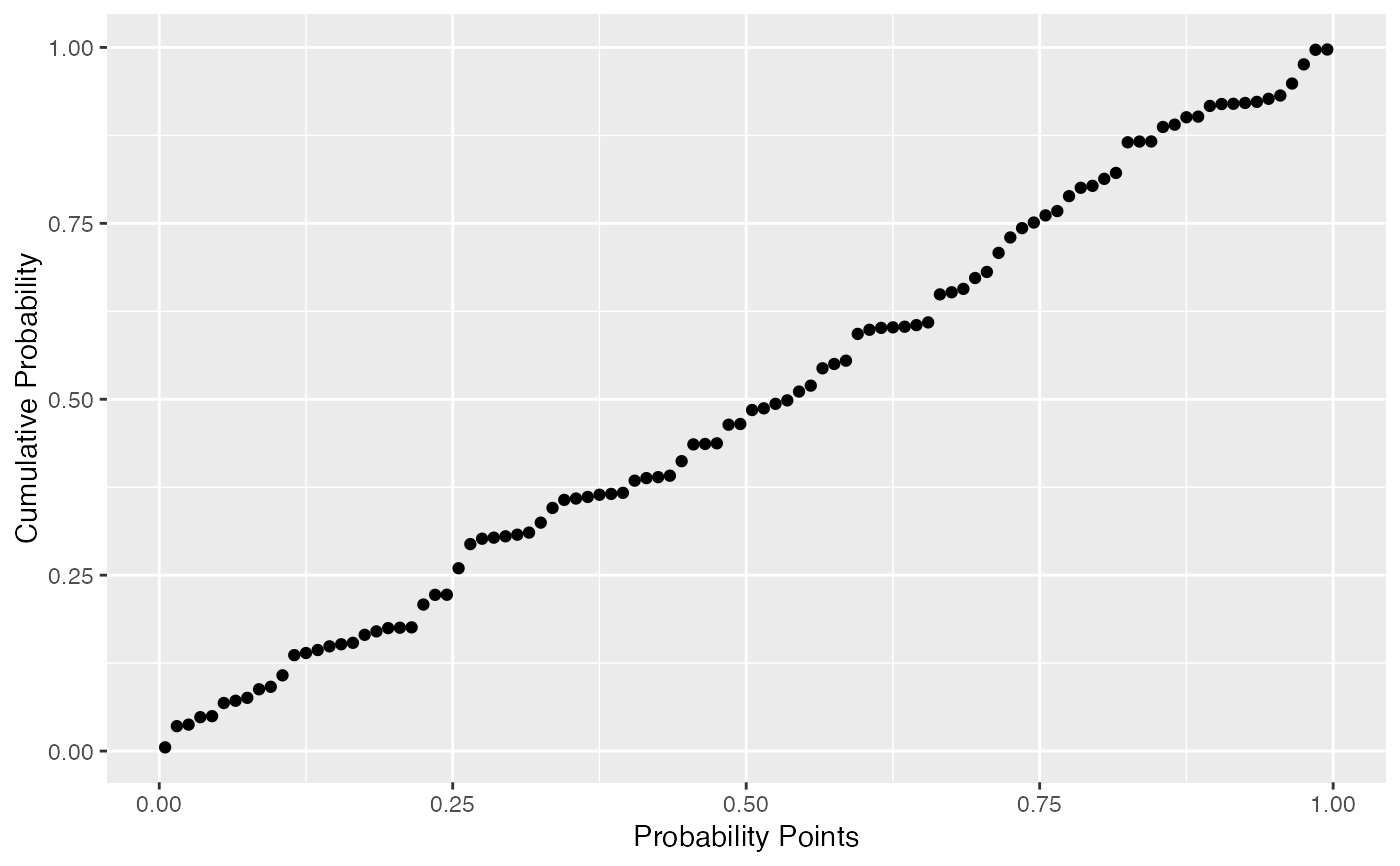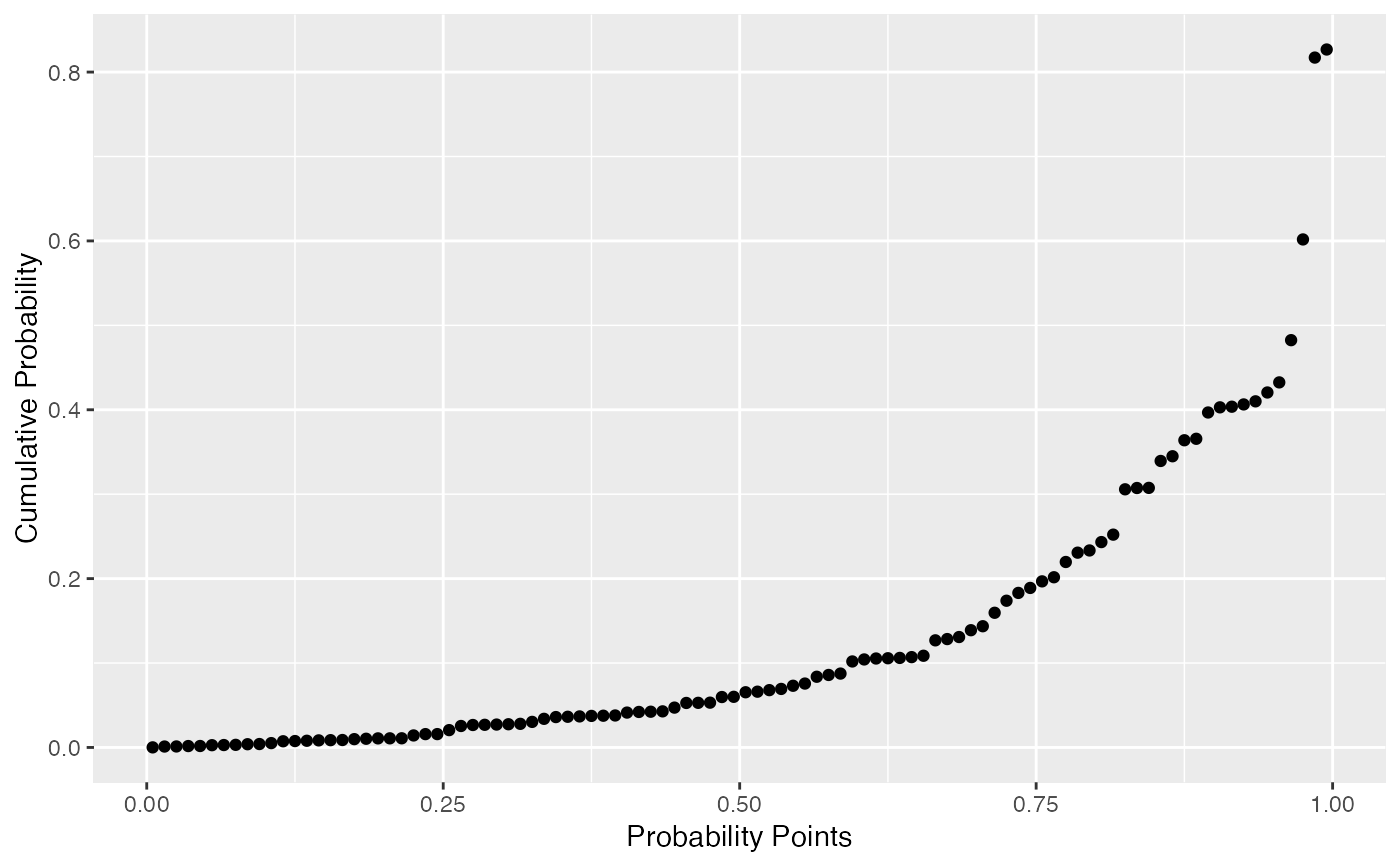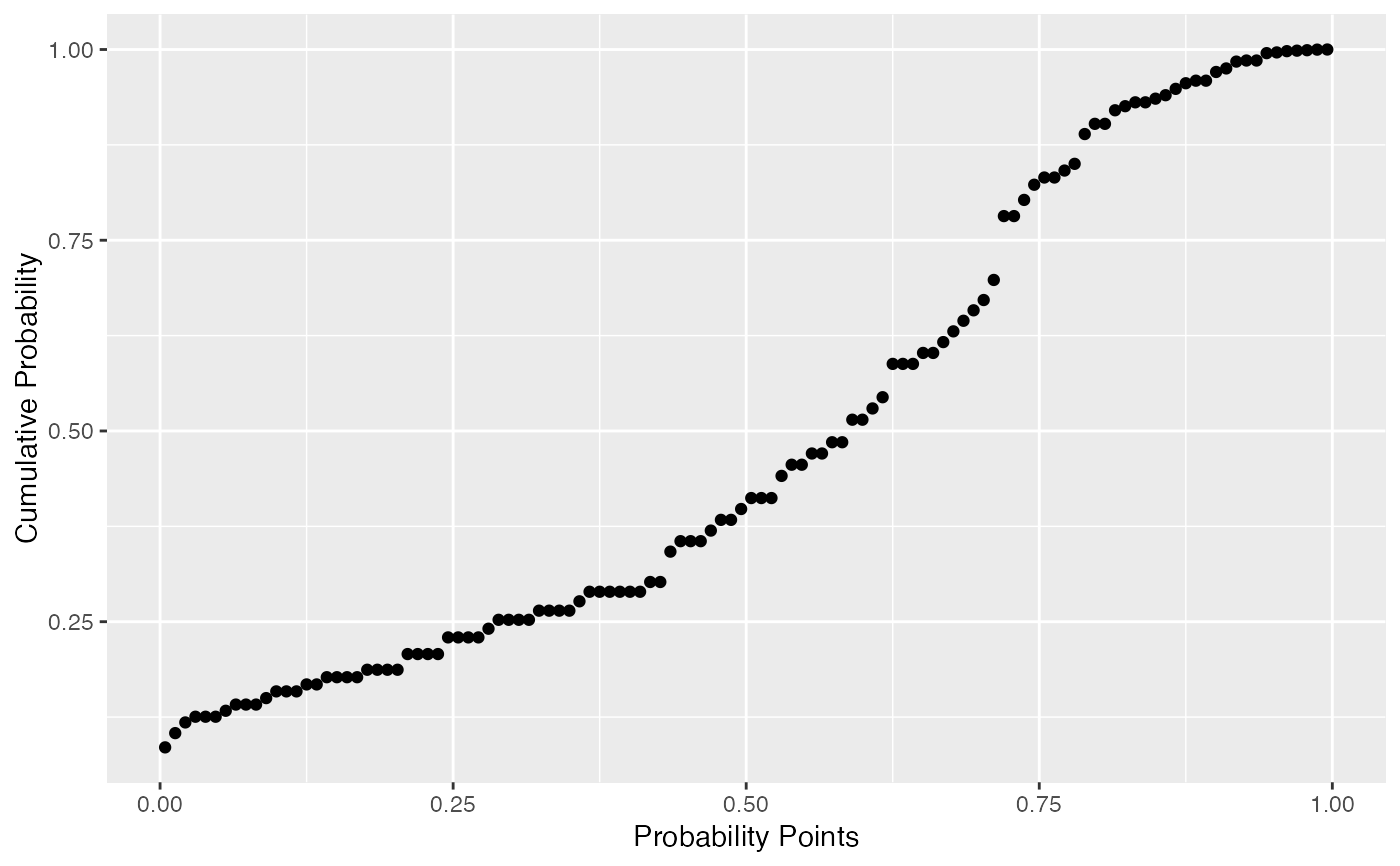Draws probability-probability points.

stat_pp_point(
mapping = NULL,
data = NULL,
geom = "point",
position = "identity",
na.rm = TRUE,
show.legend = NA,
inherit.aes = TRUE,
distribution = "norm",
dparams = list(),
detrend = FALSE,
...
)

## Arguments

mapping Set of aesthetic mappings created by aes() or aes_(). If specified and inherit.aes = TRUE (the default), it is combined with the default mapping at the top level of the plot. You must supply mapping if there is no plot mapping. The data to be displayed in this layer. There are three options: If NULL, the default, the data is inherited from the plot data as specified in the call to ggplot(). A data.frame, or other object, will override the plot data. All objects will be fortified to produce a data frame. See fortify() for which variables will be created. A function will be called with a single argument, the plot data. The return value must be a data.frame, and will be used as the layer data. A function can be created from a formula (e.g. ~ head(.x, 10)). The geometric object to use display the data Position adjustment, either as a string, or the result of a call to a position adjustment function. If FALSE, the default, missing values are removed with a warning. If TRUE, missing values are silently removed. logical. Should this layer be included in the legends? NA, the default, includes if any aesthetics are mapped. FALSE never includes, and TRUE always includes. It can also be a named logical vector to finely select the aesthetics to display. If FALSE, overrides the default aesthetics, rather than combining with them. This is most useful for helper functions that define both data and aesthetics and shouldn't inherit behaviour from the default plot specification, e.g. borders(). Character. Theoretical probability distribution function to use. Do not provide the full distribution function name (e.g., "dnorm"). Instead, just provide its shortened name (e.g., "norm"). If you wish to provide a custom distribution, you may do so by first creating the density, quantile, and random functions following the standard nomenclature from the stats package (i.e., for "custom", create the dcustom, pcustom, qcustom, and rcustom functions). List of additional parameters passed on to the previously chosen distribution function. If an empty list is provided (default) then the distributional parameters are estimated via MLE. MLE for custom distributions is currently not supported, so you must provide the appropriate dparams in that case. Logical. Should the plot objects be detrended? If TRUE, the objects will be detrended according to the default identity P-P line. This procedure was described by Thode (2002), and may help reducing visual bias caused by the orthogonal distances from P-P points to the reference line. Other arguments passed on to layer(). These are often aesthetics, used to set an aesthetic to a fixed value, like colour = "red" or size = 3. They may also be parameters to the paired geom/stat.

## Examples

# generate random Normal data
set.seed(0)
smp <- data.frame(norm = rnorm(100))

# Normal P-P plot of Normal data
gg <- ggplot(data = smp, mapping = aes(sample = norm)) +
stat_pp_point() +
labs(x = "Probability Points", y = "Cumulative Probability")
gg# Shifted Normal P-P plot of Normal data
dp <- list(mean = 1.5)
gg <- ggplot(data = smp, mapping = aes(sample = norm)) +
stat_pp_point(dparams = dp) +
labs(x = "Probability Points", y = "Cumulative Probability")
gg# Normal P-P plot of mean ozone levels (airquality dataset)
dp <- list(mean = 38, sd = 27)
gg <- ggplot(data = airquality, mapping = aes(sample = Ozone)) +
stat_pp_point(dparams = dp) +
labs(x = "Probability Points", y = "Cumulative Probability")
gg